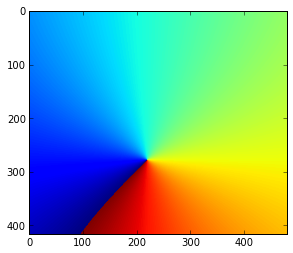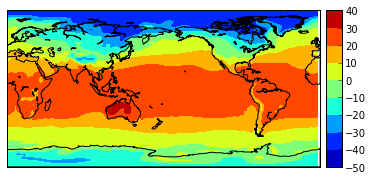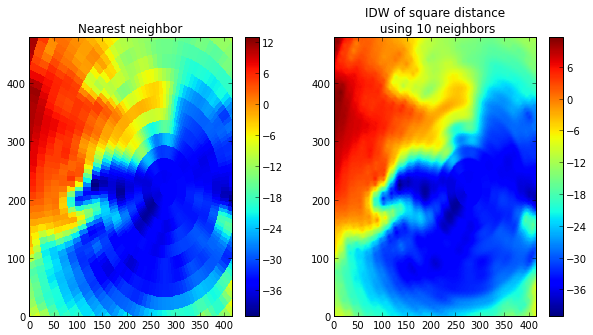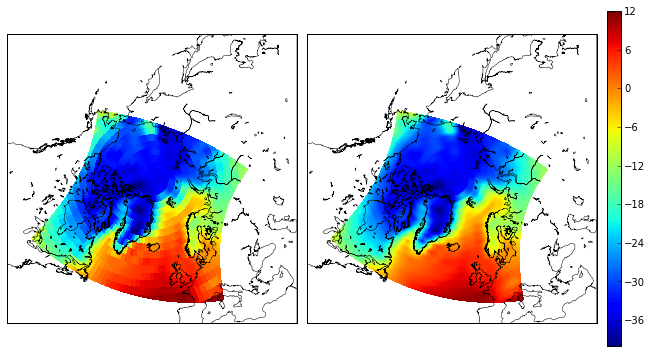# Interpolation between grids with cKDTree

Task: Interpolate data from regular to curvilinear grid

Solution: scipy.spatial.cKDTree

The problem of interpolation between various grids and projections is the one that Earth and Atmospheric scientists have to deal with sooner or later, whether for data analysis or for model validation. And when this happens it is very useful to know convnient, suitable, fast algorithms and approaches. Following the post by Nikolay Koldunov about this problem, where he proposes to deal with it using interp function from basemap package, here I present the approach using cKDTree class from scipy.spatial package. Basically this object introduces an index in k-dimensional coordinate space upon creation in order to provide very efficient querying when needed.

The algorithm I try to follow when solving this problem is: convert lat/lon coordinates to a 3D Cartesian coordinate system (here is an assumption of the spherical Earth is used), then use obtained x_s,y_s,z_s - coordinates of the source grid to construct the cKDTree object, then query the distances and indices of m closest source grid points to each target grid point. This querying is vectorized for efficinecy. Two methods of interpolation are considered here: nearest neighbour and weighting with the inverse of distance squared (i.e. $T_t = \frac{\sum_{i=1}^{m}T_{s,i}w_i}{\sum_{i=1}^{m}w_i}$, $w_i = 1/d_i^2$). The same data as in Nikolay's post will be used for the comparison sake. Netcdf4-python will be used for handling netcdf files.

Some necessary imports:

In :
%pylab inline

Populating the interactive namespace from numpy and matplotlib

In :
#for netcdf
from netCDF4 import Dataset

#for plotting
import matplotlib.pyplot as plt
from mpl_toolkits.basemap import Basemap

#for array manipulation
import numpy as np

#for interpolation
from scipy.spatial import cKDTree

import urllib2


This is the function used for converting lat/lon to x, y, z:

In :
def lon_lat_to_cartesian(lon, lat, R = 1):
"""
calculates lon, lat coordinates of a point on a sphere with
"""

x =  R * np.cos(lat_r) * np.cos(lon_r)
y = R * np.cos(lat_r) * np.sin(lon_r)
z = R * np.sin(lat_r)
return x,y,z


In :
def download_from_link(path):
import os
f_name = os.path.basename(path)
if not os.path.isfile(f_name):
remote_con = urllib2.urlopen(path)
with open(f_name, "wb") as f:


As usual we are going to use NCEP reanalysis data. This time monthly mean air temperature. Load with our helper function:

In :
download_from_link("ftp://ftp.cdc.noaa.gov/Datasets/ncep.reanalysis.derived/surface/air.mon.mean.nc")


We also need coordinates of the curvilinear grid:

In :
download_from_link("https://www.dropbox.com/s/9xzgyjs08zyuwzw/curv_grid.nc")


Let's have a look at our curvilinear grid:

In :
fc = Dataset('curv_grid.nc')
lon_curv = fc.variables['xc'][0,:,:]
lat_curv = fc.variables['yc'][0,:,:]


Distribution of longitudes, for example, looks like this:

In :
plt.imshow(lon_curv);This is grid from the regional Arctic Ocean model with 40 km resolution.

Now load NCEP data (only first time step), that have 2.5 degree resolution:

In :
fr = Dataset('air.mon.mean.nc')

In :
air = fr.variables['air'][0,:,:]
lat = fr.variables['lat'][:]
lon = fr.variables['lon'][:]


First look at the data:

In :
lon2d, lat2d = np.meshgrid(lon, lat)
b = Basemap(lon_0=180) #This defines the center of the map, 180 since longitudes go from 0 to 360
x, y = b(lon2d, lat2d)
b.contourf(x, y, air)
b.drawcoastlines()
b.colorbar();When all the necessary inputs are read in memory, convert lat/lon to the Cartesian coordinate reference system (CRS):

In :
xs, ys, zs = lon_lat_to_cartesian(lon2d.flatten(), lat2d.flatten())
xt, yt, zt = lon_lat_to_cartesian(lon_curv.flatten(), lat_curv.flatten())


Create cKDTree object to represent source grid.

In :
tree = cKDTree(zip(xs, ys, zs))


Nearest neighbour interploation:

In :
#find indices of the nearest neighbors in the flattened array
d, inds = tree.query(zip(xt, yt, zt), k = 1)
#get interpolated 2d field
air_nearest = air.flatten()[inds].reshape(lon_curv.shape)


Interpolate using inverse distance weighting, using 10 nearest neighbours (k=10):

In :
d, inds = tree.query(zip(xt, yt, zt), k = 10)
w = 1.0 / d**2
air_idw = np.sum(w * air.flatten()[inds], axis=1) / np.sum(w, axis=1)
air_idw.shape = lon_curv.shape


Now let us plot the results from these two approaches

In :
fig = plt.figure(figsize=(10,5))
subplot(121)
plt.pcolormesh(air_nearest.transpose())
plt.xlim([0, air_nearest.shape])
plt.ylim([0, air_nearest.shape])
plt.colorbar()
plt.title("Nearest neighbor")

subplot(122)
plt.pcolormesh(air_idw.transpose())
plt.colorbar()
plt.xlim([0, air_nearest.shape])
plt.ylim([0, air_nearest.shape])
plt.title("IDW of square distance \n using 10 neighbors");In :
m = Basemap(projection='npstere',boundinglat=40,lon_0=-20,resolution='l')
x, y = m(lon_curv, lat_curv)

In :
fig = plt.figure(figsize=(11,6))

from matplotlib.gridspec import GridSpec
gs = GridSpec(1,3, width_ratios=[1,1, 0.05], wspace = 0.05)

m.drawcoastlines(linewidth=0.5)
cs = m.pcolormesh(x,y,air_nearest, vmin =-40, vmax=12, ax = ax)